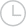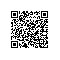# numpy如何比我的Fortran例程快得多？-问答-阿里云开发者社区-阿里云

## numpy如何比我的Fortran例程快得多？保持可爱mmm2020-02-08 14:35:4571

integer gridsize,unit,j real mini,maxi double precision mean

gridsize=512 unit=40 open(unit=unit,file='T.out',status='old',access='stream',& form='unformatted',action='read') read(unit=unit) tmp mini=tmp maxi=tmp mean=tmp do j=2,gridsize3 read(unit=unit) tmp if(tmp>maxi)then maxi=tmp elseif(tmp<mini)then mini=tmp end if mean=mean+tmp end do mean=mean/gridsize3 close(unit=unit) 我使用的计算机上的每个文件大约需要25秒。那让我感到震惊，因为它相当长，所以我继续在Python中执行以下操作：

``````import numpy

mmap=numpy.memmap('T.out',dtype='float32',mode='r',offset=4,\
shape=(512,512,512),order='F')
mini=numpy.amin(mmap)
maxi=numpy.amax(mmap)
mean=numpy.mean(mmap)
``````

numpy怎么这么快？我的意思是，您必须查看数组的每个条目才能找到这些值，对吗？我是否在我的Fortran例程中做了一些非常愚蠢的事情，以使其花费了更长的时间？ 问题来源于stack overflow取消 提交回答

• 保持可爱mmm
2020-02-08 14:36:01

您的Fortran实施存在两个主要缺点：

您将IO和计算混合在一起（并逐个条目从文件中读取）。 您不使用向量/矩阵运算。 此实现确实执行与您相同的操作，并且在我的机器上速度提高了20倍：

program test integer gridsize,unit real mini,maxi,mean real, allocatable :: tmp (:,:,:)

gridsize=512 unit=40

allocate( tmp(gridsize, gridsize, gridsize))

open(unit=unit,file='T.out',status='old',access='stream',& form='unformatted',action='read') read(unit=unit) tmp

close(unit=unit)

mini = minval(tmp) maxi = maxval(tmp) mean = sum(tmp)/gridsize**3 print *, mini, maxi, mean

end program 想法是一次性将整个文件读入一个数组tmp。然后，我可以使用的功能MAXVAL，MINVAL和SUM在阵列上直接。

对于精度问题：只需使用双精度值，然后按以下方式即时进行转换

mean = sum(real(tmp, kind=kind(1.d0)))/real(gridsize**3, kind=kind(1.d0)) 仅略微增加了计算时间。我尝试按元素和分片方式执行操作，但这仅增加了默认优化级别所需的时间。

在处-O3，逐元素加法的性能比数组运算好〜3％。在我的机器上，双精度和单精度运算之间的差异小于2％-平均而言（单个运算的偏差要大得多）。

这是使用LAPACK的非常快速的实现：

program test integer gridsize,unit, i, j real mini,maxi integer :: t1, t2, rate real, allocatable :: tmp (:,:,:) real, allocatable :: work(:) ! double precision :: mean real :: mean real :: slange

call system_clock(count_rate=rate) call system_clock(t1) gridsize=512 unit=40

allocate( tmp(gridsize, gridsize, gridsize), work(gridsize))

open(unit=unit,file='T.out',status='old',access='stream',& form='unformatted',action='read') read(unit=unit) tmp

close(unit=unit)

mini = minval(tmp) maxi = maxval(tmp)

! mean = sum(tmp)/gridsize3 ! mean = sum(real(tmp, kind=kind(1.d0)))/real(gridsize3, kind=kind(1.d0)) mean = 0.d0 do j=1,gridsize do i=1,gridsize mean = mean + slange('1', gridsize, 1, tmp(:,i,j),gridsize, work) enddo !i enddo !j mean = mean / gridsize**3

print *, mini, maxi, mean call system_clock(t2) print *,real(t2-t1)/real(rate)

end program 这SLANGE在矩阵列上使用单精度矩阵1-范数。运行时间甚至比使用单精度数组函数的方法快-并且没有显示精度问题。

0 0使用钉钉扫一扫加入圈子
+ 订阅